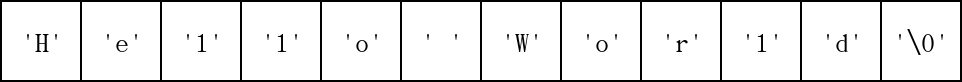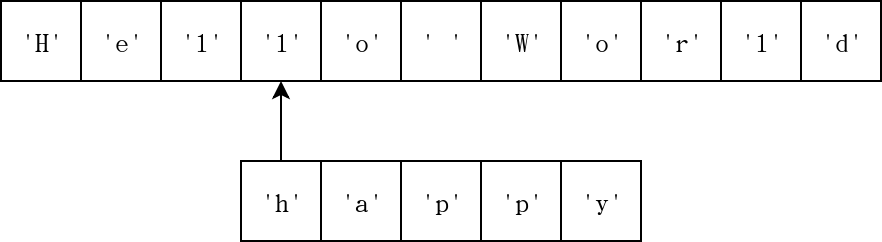#[C 語言] 程式設計教學：如何使用 C 字串 (String)

## C 語言的字串方案

• 固定寬度字元陣列
• 字元陣列 (character array)
• 寬字元陣列 (wide character array)：使用 wchar.h 函式庫
• 多字節編碼 (multibyte encodings)，像是 Big5 (大五碼) 或 GB2312 等
• 統一碼 (Unicode)：包括 UTF-8、UTF-16、UTF-32 等

``````#include <locale.h>
#include <wchar.h>

int main(void)
{
// Trick to print multibyte strings.
setlocale(LC_CTYPE, "");

wchar_t *s = L"你好，世界";
printf("%ls\n", s);

return 0;
}``````

## C 字串微觀## 計算 C 字串長度

``````#include <assert.h>                  /*  1 */
#include <stddef.h>                  /*  2 */
#include <string.h>                  /*  3 */

int main(void)                       /*  4 */
{                                    /*  5 */
char s[] = "Hello";              /*  6 */

size_t sz = 0;                   /*  7 */

for (size_t i = 0; s[i]; i++) {  /*  8 */
sz++;                        /*  9 */
}                                /* 10 */

assert(sz == strlen(s));         /* 11 */

return 0;                        /* 12 */
}                                    /* 13 */``````

## 複製 C 字串

``````#include <assert.h>                                        /*  1 */
#include <stddef.h>                                        /*  2 */
#include <stdio.h>                                         /*  3 */
#include <stdlib.h>                                        /*  4 */
#include <string.h>                                        /*  5 */

int main(void)                                             /*  6 */
{                                                          /*  7 */
char s[] = "Hello World";                              /*  8 */

size_t sz_s = strlen(s);                               /*  9 */
size_t sz = sz_s + 1;  /* Add trailing zero. */        /* 10 */

char *out = malloc(sz * sizeof(char));                 /* 11 */
if (!out) {                                            /* 12 */
fprintf(stderr, "Failed to allocate C string\n");  /* 13 */
return 1;                                          /* 14 */
}                                                      /* 15 */

for (size_t i = 0; i < sz_s; i++) {                    /* 16 */
out[i] = s[i];                                     /* 17 */
}                                                      /* 18 */

out[sz-1] = '\0';                                      /* 19 */

assert(0 == strcmp(out, "Hello World"));               /* 20 */

free(out);                                             /* 21 */

return 0;                                              /* 22 */
}                                                          /* 23 */``````

## 相接兩個 C 字串

``````#include <assert.h>                            /*  1 */
#include <stdio.h>                             /*  2 */
#include <stdlib.h>                            /*  3 */
#include <string.h>                            /*  4 */

int main(void) {                               /*  5 */
char s_a[] = "Hello ";                     /*  6 */
char s_b[] = "World";                      /*  7 */

size_t sz_a = strlen(s_a);                 /*  8 */
size_t sz_b = strlen(s_b);                 /*  9 */
size_t sz = sz_a + sz_b + 1;               /* 10 */

char *out = malloc(sz * sizeof(char));     /* 11 */
if (!out) {                                /* 12 */
fprintf(stderr,                        /* 13 */
"Failed to allocate C string\n");  /* 14 */
return 1;                              /* 15 */
}                                          /* 16 */

for (size_t i = 0; i < sz_a; i++) {        /* 17 */
out[i] = s_a[i];                       /* 18 */
}                                          /* 19 */

for (size_t i = 0; i < sz_b; i++) {        /* 20 */
out[i+sz_a] = s_b[i];                  /* 21 */
}                                          /* 22 */

out[sz-1] = '\0';                          /* 23 */

assert(strcmp(out, "Hello World") == 0);   /* 24 */

free(out);                                 /* 25 */

return 0;                                  /* 26 */
}                                              /* 27 */``````

## 檢查兩個 C 字串是否相等

``````#include <assert.h>               /*  1 */
#include <stdbool.h>              /*  2 */

int main(void)                    /*  3 */
{                                 /*  4 */
char a[] = "happy";           /*  5 */
char b[] = "happy hour";      /*  6 */

bool is_equal = true;         /*  7 */

char *p = a;                  /*  8 */
char *q = b;                  /*  9 */
while (*p && *q) {            /* 10 */
/* Unequal character. */  /* 11 */
if (*p != *q) {           /* 12 */
is_equal = false;     /* 13 */
goto END;             /* 14 */
}                         /* 15 */

p++; q++;                 /* 16 */
}                             /* 17 */

/* Unequal string length. */  /* 18 */
if (*p != *q) {               /* 19 */
is_equal = false;         /* 20 */
goto END;                 /* 21 */
}                             /* 22 */

END:                              /* 23 */
assert(false == is_equal);    /* 24 */

return 0;                     /* 25 */
}                                 /* 26 */``````

## 尋找子字串``````#include <assert.h>                                            /*  1 */
#include <stdbool.h>                                           /*  2 */

int main(void)                                                 /*  3 */
{                                                              /*  4 */
/* Original string. */                                     /*  5 */
char s[] = "The quick brown fox jumps over the lazy dog";  /*  6 */
/* Substring. */                                           /*  7 */
char ss[] = "lazy";                                        /*  8 */

bool is_found = false;                                     /*  9 */

char *p = s;                                               /* 10 */
while (*p) {                                               /* 11 */
bool temp = true;                                      /* 12 */

char *q = p;                                           /* 13 */
char *r = ss;                                          /* 14 */
while (*q && *r) {                                     /* 15 */
if (*q != *r) {                                    /* 16 */
temp = false;                                  /* 17 */
break;                                         /* 18 */
}                                                  /* 19 */

q++; r++;                                          /* 20 */
}                                                      /* 21 */

if (!(*r)) {                                           /* 22 */
is_found = true;                                   /* 23 */
break;                                             /* 24 */
}                                                      /* 25 */

p++;                                                   /* 26 */
}                                                          /* 27 */

assert(is_found);                                          /* 28 */

return 0;                                                  /* 29 */
}                                                              /* 30 */``````

## 繼續深入